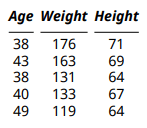## Create Tables from MATLAB Tables

To create a DOM API table from a MATLAB® table, use an `mlreportgen.dom.MATLABTable` object. The DOM representation of a MATLAB table has the structure of a DOM formal table. See Create Formal Tables. The `MATLABTable` header contains the column names from the MATLAB table. The `MATLABTable` body contains the rows and elements from the MATLAB table.

If the table in MATLAB has row names, the first column of the `MATLABTable` object contains the row names. In MATLAB, the row name column does not have a label. In the `MATLABTable` object, you can specify a label for the row name column by changing the content of the first element of the header. You can specify a line under the row name column label by setting the `RowNamesRule` property of the `MATLABTable` object to `true`.

For information about other types of tables that you can create in reports, see Choose Type of Table to Create.

### Create a Table from a MATLAB Table

This example creates a DOM table from a MATLAB table that has row names. The example generates two reports that include the DOM table. In the first report, the row names column of the table does not have a label. In the second report, the row names column has a label.

Create a MATLAB table that shows the age, weight, and height of patients. Use the `RowNames` option to identify each row by the last name of the patient.

```LastName = {'Sanchez';'Johnson';'Lee';'Diaz';'Brown'}; Age = [38;43;38;40;49]; Weight = [176;163;131;133;119]; Height = [71;69;64;67;64]; mltable = table(Age,Weight,Height,'RowNames',LastName)```
```mltable=5×3 table Age Weight Height ___ ______ ______ Sanchez 38 176 71 Johnson 43 163 69 Lee 38 131 64 Diaz 40 133 67 Brown 49 119 64 ```

The MATLAB table has five rows and three columns. The row names are not part of the table. They are stored in a property of the table.

Create an `mlreportgen.dom.MATLABTable` object from the `MATLAB` table.

```import mlreportgen.dom.* mltableObj = MATLABTable(mltable);```

Create a document and append the `MATLABTable` object to the document. Close and view the document.

```d = Document('MyMATLABTable1','docx'); append(d,mltableObj); close(d); rptview(d);```

Here is the table in the generated report:The DOM table is a formal table, which has a header and a body. The table body has five rows and four columns. The first column consists of the MATLAB table row names.

Generate the report again, this time with a label for the column of row names. To specify the label, replace the empty text in the first entry of the table header row with the label text. To draw a line under the label, set the `RowNamesRule` property of the `MATLABTable` object to `true`.

```LastName = {'Sanchez';'Johnson';'Lee';'Diaz';'Brown'}; Age = [38;43;38;40;49]; Weight = [176;163;131;133;119]; Height = [71;69;64;67;64]; mltable = table(Age,Weight,Height,'RowNames',LastName); import mlreportgen.dom.* mltableObj = MATLABTable(mltable); th = mltableObj.Header; thentry11 = entry(th,1,1); thentry11.Children(1).Children(1).Content = 'Names'; mltableObj.RowNamesRule = true; d = Document('MyMATLABTable2','docx'); append(d,mltableObj); close(d); rptview(d);```

Here is the table in the generated report:### Format a Table Created from a MATLAB Table

By default, a table generated from a `MATLABTable` object is formatted to look like a table in MATLAB. To customize the appearance of the table, you can use the same approaches that you use for other types of tables:

• Update the default style in the style sheets of the default HTML, Word, or PDF template. The default `StyleName` of a `MATLABTable` object is `"rgMATLABTable"`.

• Set the `StyleName` property to a custom style.

• Use format properties or format objects with the table or a section of the table.

See Format Tables.

#### Format the Sections of a MATLAB `Table`

This example shows how to format the sections of a DOM table that is created from a MATLAB table.

This example makes the column headings italic.To access the header, use the `Header` property of the `mlreportgen.dom.MATLABTable` object. The example adds an `mlreportgen.dom.Italic` format object to the `Style` property of the `mlreportgen.dom.TableHeader` object that represents the header.

```import mlreportgen.dom.* d = Document('myMATLABTable','pdf'); Age = [38;43;38;40;49]; Height = [71;69;64;67;64]; Weight = [176;163;131;133;119]; mltable = table(Age,Weight,Height); mltableObj = MATLABTable(mltable); mltableObj.Header.Style = [mltableObj.Header.Style {Italic(true)}]; append(d,mltableObj); close(d); rptview(d);```

Format the Table Body

This example makes the entries of the first row of the table body blue.To access the table body, use the `Body` property of the `mlreportgen.dom.MATLABTable` object. To access a row of the body, use the `row` method of the `mlreportgen.dom.TableRow` object that represents the row. The example adds an `mlreportgen.dom.Color` format object to the S`tyle` property of the `TableRow` object that represents the first row.

```import mlreportgen.dom.* d = Document('myMATLABTable','pdf'); Age = [38;43;38;40;49]; Height = [71;69;64;67;64]; Weight = [176;163;131;133;119]; mltable = table(Age,Weight,Height); mltableObj = MATLABTable(mltable); tbody = mltableObj.Body; row1 = row(tbody,1); row1.Style = [row1.Style {Color('blue')}]; append(d,mltableObj); close(d); rptview(d);```